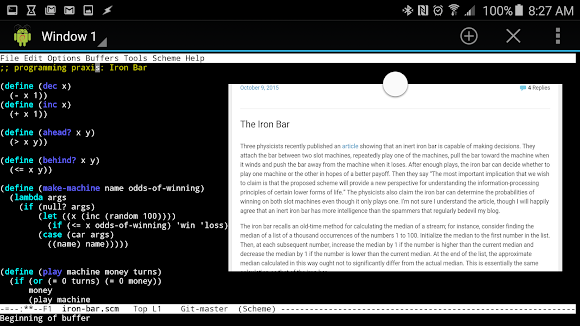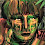## Wednesday, October 14, 2015

### Smart as a box of hammers: using a physical object to make smart decisions

The ProgrammingPraxis Iron Bar exercise was too intriguing not to play with. It references this article which ponders if a rigid object (say, an iron bar) can be used to make decisions. Specifically, and this is where they truly sucked me, can it determine which is the optimal slot machine to play.

The algorithm at play here is as simple as could be:

They attach the bar between two slot machines, repeatedly play one of the machines, pull the bar toward the machine when it winds and push the bar away from the machine when it loses. After enough plays, the iron bar can decide whether to play one machine or the other in hopes of a better payoff.

Does it really work that way? Let's find out.First, I need an implementation of a slot machine, and one that's biased at that. Here you go:

```(define (dec x)
(- x 1))
(define (inc x)
(+ x 1))

(> x y))

(define (behind? x y)
(<= x y))

(define (make-machine name odds-of-winning)
(lambda args
(if (null? args)
(let ((x (inc (random 100))))
(if (<= x odds-of-winning) 'win 'loss))
(case (car args)
((name) name)))))
```

make-machine is called with an odds-of-winning argument, which determines what percentage of the time it pays off. For this experiment, here's my three machines:

```(define m1 (make-machine 'm1 100))
(define m2 (make-machine 'm2 10))
(define m3 (make-machine 'm3 13))
```

m1 is a sort of control, always causing a winner. m2 and m3 and cause a win 10% and 13% of the time respectively. These values weren't thought particularly through, other than the fact that 10% and 13% aren't *that* different.

And here's a function that plays these machines. You drop in a certain dollar amount and a number of turns, and the result is how much money you have at the end.

```(define (play machine money turns)
(if (or (= 0 turns) (= 0 money))
money
(play machine
(+ (dec money)
(if (eq? (machine) 'win) 10 0))
(dec turns))))
```

Here's an example of a \$100, 100 turn session with each of the machines:

```(play m1 100 100)   ; => 1000
(play m2 100 100)   ; => 80
(play m3 100 100)   ; => 130
```

Note how m2 didn't come out ahead, whereas m3 did. However, one session won't tell you all that much, which is where the trial function comes in. It runs count number of play sessions and returns a pair: the number of winning session and the number of losing sessions.

```(define (trial count machine money turns)
(let loop ((count count) (outcome (cons 0 0)))
(cond ((= 0 count) outcome)
(else
(let ((result (play machine money turns)))
(loop (dec count)
(cons
(if (ahead? result money) (inc (car outcome)) (car outcome))
(if (behind? result money) (inc (cdr outcome)) (cdr outcome)))))))))
```

Let's see that in action:

```(trial 100 m1 100 100)   ; => '(100 . 0)
(trial 1000 m2 100 100)  ; => '(425 . 575)
(trial 1000 m3 100 100)  ; => '(764 . 236)
```

For m1 we see that 100 out of 100 times, it was a winner. That's good, as the odds of winning this machine are 100%. m2 and m3 are where things get interesting. Note how m2 one 425 times out of 1,000, whereas m3 won 764 times out of 1,000. This shows that m3 is really biased towards winning, whereas m2 is not. Can you have losing session with m3? Sure, it happened 236 times. And can you have a winning session with m2? Of course, it happened 425 times. But overall, m3 is the far better machine to play.

At this point, our iron bar has just been sitting on the shelf. Nothing in the above code has anything to do with decision making, it just shows us what we already know: the 13% machine pays off more than the 10% machine. (Though as a programmer, it sure is nice to see things work as you expect).

OK, let's bust out the iron bar and put it to work. Using the above algorithm, can the bar determine that m3 is a better deal than m2? This function, observe is designed to do just that:

```(define (observe count the-m1 the-m2)
(let loop ((m1 the-m1) (m2 the-m2) (count count) (offset 0) (dir -1))
(if (= 0 count)
(cons (cond ((= 0 offset) '??)
((> offset 0) (the-m2 'name))
(else (the-m1 'name)))
offset)
(let ((result (m1)))
(loop m2 m1
(dec count)
(+ offset
(* (if (eq? result 'win) 1 -1) dir))
(* dir -1))))))
```

Note that observe doesn't care about money or sessions. It's just looking over the gambler's shoulder and nudging the bar one way or the other based on the outcome. At the end of count observations, it reports which machine is optimal along with the offset of the bar. The larger the offset, the more confident you can be that the machine it selected is a winner.

Let's compare m1 and m2:

```(observe 100 m1 m2)      ; => '(m1 . -96)
```

After watching these two machines, m1 is selected with an offset of -96. This means that the bar is quite convinced that m1 is the ideal machine even though m2 occasionally won. Considering m1 pays off 100% of the time, this is good news, though hardly shocking.

Now to the fun part: m2 vs m3:

```(observe 10 m2 m3)       ; => '(m3 . 4) '(m2 . -2) '(?? . 0)
(observe 100 m2 m3)      ; => '(m3 . 8) '(m2 . -2) '(m3 . 12)
(observe 1000 m2 m3)     ; => '(m3 . 48) '(m3 . 34) '(m3 . 58)
```

Running 10 observations 3 times gives pretty inconclusive results: m3 is preferred, then m2 then nobody is selected.

Things aren't much better after 100 observations. m3 seems to be the preferred choice, but m2 still looks better sometimes, though admittedly not by much (-2 is just a slight preference for m2).

For 1,000 observations, our bar really performs: it correctly selects m3 every time and does so with significant confidence.

So can an iron bar make decisions? As the above shows, definitely.

In some respects, this seems totally obvious. In fact, even now I won't be shocked if someone leaves a comment letting me know I was punk'd. Of course moving a bar after every win and loss is going to show you a preference, it's like keeping a running total and looking back to that total at the end of the sequence. What's the big deal?

On the other hand, this is absolutely brilliant. The fact that simple mechanics can translate to cognitive behavior is amazing, and means that even the simplest forms of life can "think." I could absolutely see how this would play into evolutionary biology, and how species could use this behavior as a way to survive.

Find all the code above here to read and run.

Developed with racket, emacs and GNUroot on my Galaxy Note5.

#### 1 comment:

1.I first got interested in this kind of thing when I read A. K. Dewdney's famous Scientific American article on the "spaghetti sort." Here's another: water can solve a maze. Tip a maze so that the entrance is higher than the exit, and pour water into the maze. Eventually, the current will flow from the entrance to the exit.